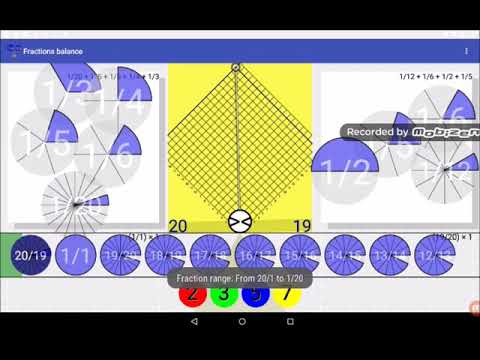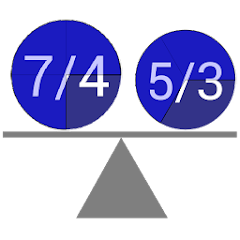# Fractions Balance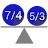1K+Everyone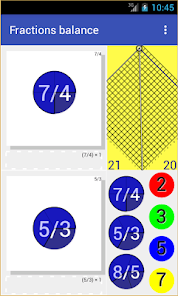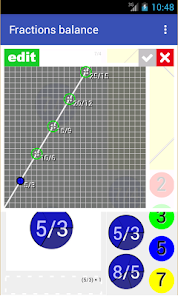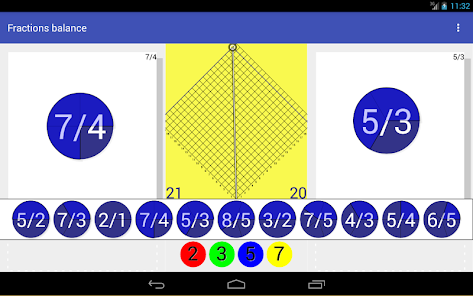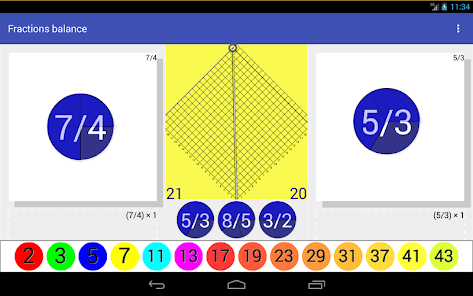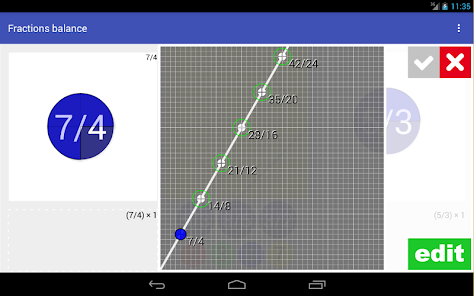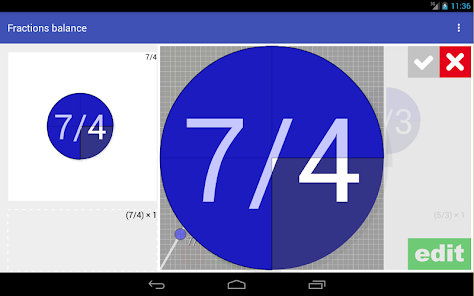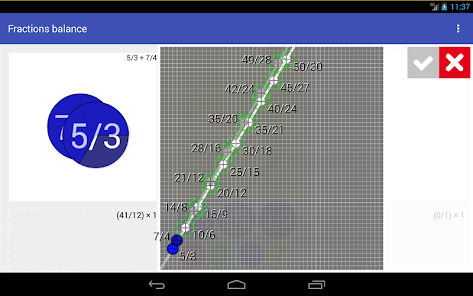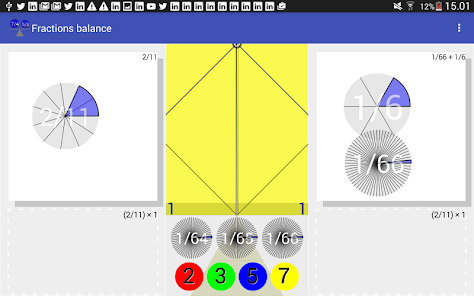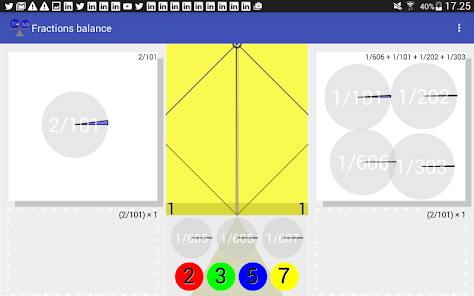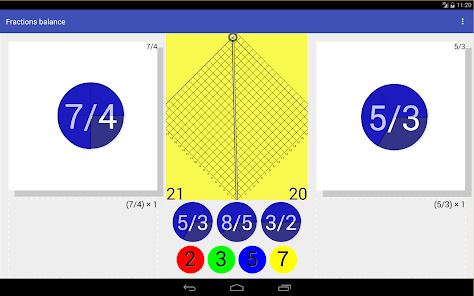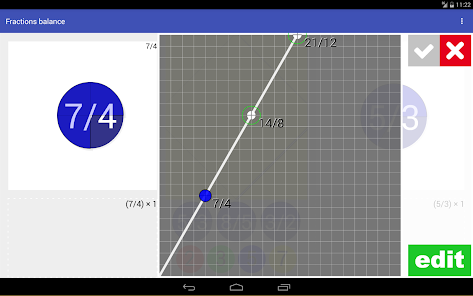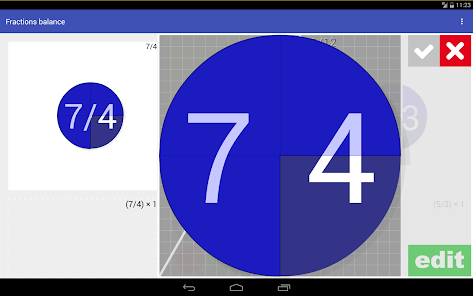Pan balance: Fractions

Balance fractions. (Fractions division)
Equivalent fractions.
Reduce fractions.
Weigh fractions.
Balance a fractions balance scale, multiplying fractions by prime numbers. (division of fractions)
Live fulcrum and balance scale.

Usually in elementary mathematics, teaching pan scale balances are used only for display them at the time of equilibrium, to verify that two quantities are equivalent.
In this case, apart of this use, it is also useful imbalance in the balance.
This imbalance, when the ratio is proportional to the ratio of content of the dishes, is also the result of the division.
In this case, the result of the division of fractions.
To view it, you can multiply the contents of each dish, until the balance is obtained, the numbers for which has multiplied each dish are in turn the result fraction of the division.
The program only multiply by prime numbers, because any number can be built with them.

(This app is the logical continuation of the work done in "Mathcats balance" of MATHCATS).

FRACTIONS BALANCE IDEAS:
Experiments with Fractions balance:

Video 1: Comparing: 1/2 + 1/3 + 1/6
with: 1/3 + 1/5 + 1/6 + 1/8 + 1/10 + 1/12
and: 1/3 + 1/4 + 1/5 + 1/8 + 1/12

Registered at Math Tools (Math Forum)
http://mathforum.org/mathtools/tool/273014/

From nummolt.com
Nummolt apps:
"Mathematics is the toughest toy. However mischievous a child may be, will never be able to break them".
www.nummolt.com
Updated on
Oct 4, 2020

## Data safety

Safety starts with understanding how developers collect and share your data. Data privacy and security practices may vary based on your use, region, and age. The developer provided this information and may update it over time.No data shared with third parties